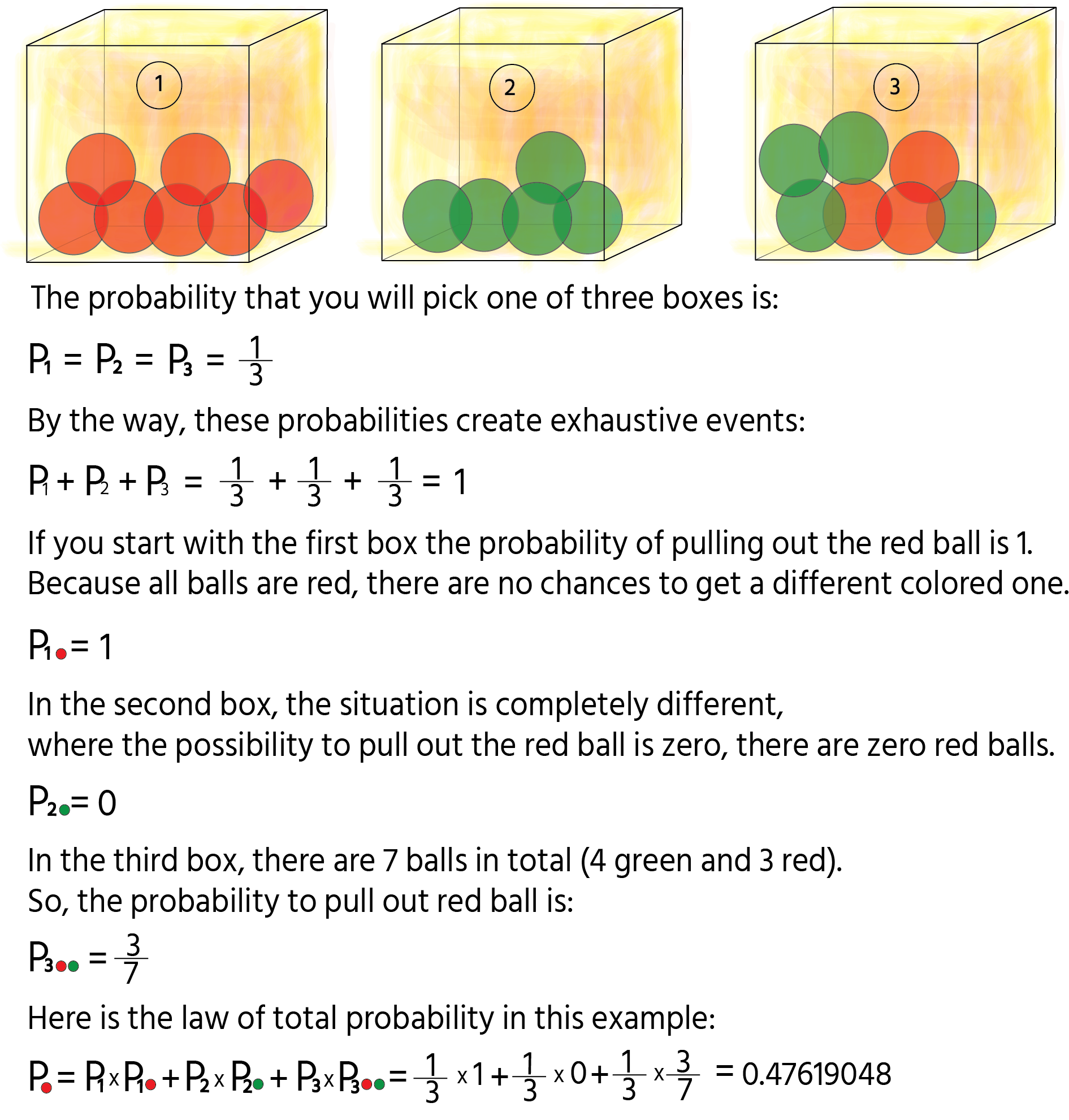Course Content

Probability TheoryLaw of Total Probability

It is time to gain insight into theoretical terms! Let's start.

The first crucial term is exhaustive events.

What is it?

This term describes when at least one event must happen.

Example:

The simplest and the most evident example is tossing a coin. The probability of receiving head or tail is 50%, but together they result in 100%(all outcomes). In other words, the events are exclusive.

Moving on, let us look at another term i.e. law of total probability.

To understand better, it is better to solve one task.

Example:

Imagine that we have three boxes. The first one contains 7 red balls, the second one contains 5 green balls and the third one contains 4 green balls and 3 red balls. Calculate the probability that we will randomly pull out red ball.So, the result is 0.47619048, to find it we should multiply the probability to pick a specific box by the probability to pull out the box from this column.#### You have two baskets the first one contains 3 cats' toys and 7 dog's (10 toys), the second one contains 6 cat's toys and 14 dog's (20 toys). The probability to choose one of the basket is 50% or 0.5. Calculate the probability to get cats' toy. Place items in the correct order.

Probability = 3/10
___
0.5
___
6/20
___
___

Click or drag`n`drop items and fill in the blanks0.5**+Section 2.

Chapter 6